# Texas Go Math Grade 5 Lesson 10.4 Answer Key Make Graphs

Refer to our Texas Go Math Grade 5 Answer Key Pdf to score good marks in the exams.
Test yourself by practicing the problems from Texas Go Math Grade 5 Lesson 10.4 Answer Key Make Graphs.

## Texas Go Math Grade 5 Lesson 10.4 Answer Key Make Graphs

Unlock the Problem

An airport taxi uses the rule f= m + 2 to calculate the fare for passengers.
The number of miles m is the input and the output is the fare f in dollars.
Graph the pattern.STEP 1: Label the horizontal number line to show that the input is number of miles.
Label the vertical number line to show that the output is the fare.

STEP 2 Write a number pair and graph it.
For a trip that is 2 miles, the fare will be $_______. The number pair is (2, _4_). Move right _______ units. Then move up _______ units and draw a point to represent the number pair. STEP 3: Graph several number pairs. Move right __________ units. (__________, __________) Move up __________ units. Move right __________ units. (__________, __________) Move up __________ units. Move right __________ units. (__________, __________) Move up __________ units. Math Talk Mathematical Processes Explain what each number in the number pair represents. Answer: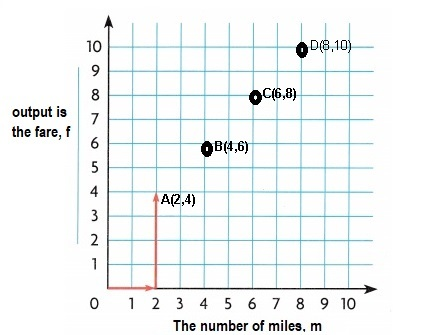The numbers on x – axis is number of miles m and y- axis is the output fare as f =m +$2, so the number pair
represented are A(2,4), B(4,6), C(6,8) and D(8,10),

Explanation:
STEP 1: Labeled the horizontal number line to show that the input is number of miles m,
Labeled the vertical number line to show that the output is the fare f,
STEP 2: Given An airport taxi uses the rule f= m + 2 to calculate the fare for passengers.
Wrote a number pair and graphed  it as shown above,
For a trip that is 2 miles, the fare will be f = 2 + $2 =$4,
so the number pair is (2,4), Moved right 2 units. Then moved up 4 units and
drawn a point to represent the number pair as A(2,4),
STEP 3: Graphed several number pairs as
Moved right 2 more units from 2 we get 4
then moved up 4 + $2 = 6 units, So we get B(4,6), Moved right 2 more units from 4 we get 6 then moved up 6 +$2 = 8 units, we get C(6, 8),
Moved right 2 more units from 6 we get 8
then moved up 8 + $2 = 10 units, So we get B(8,10), The numbers in the number pair represents A(2,4), B(4,6), C(6,8) and D(8,10) respectively. Example Graph a multiplicative pattern. Celina is counting the number of wheels on tricycles. The rule is w = 3t, where t is the number of tricycles and w is the number of wheels. Use the rule to show a pattern on the graph.STEP 1: Label the horizontal number line to show the input is the number of tricycles. Label the vertical number line to show the output is the number of __________. STEP 2: Write a number pair and graph it. The input _________ times 3 is equal to the output __________. The number pair is (__________, __________) STEP 3; Graph several number pairs. Move right __________ units. (__________, __________) Move up __________ units. Move right __________ units. (__________, __________) Move up __________ units. Move right __________ units. (__________, __________) Move up __________ units. Answer:The numbers on x – axis is number of tricycles t and y- axis is the output number of wheels w as w =3t, so the number pair represented are A(1,3), B(2,6), C(3,9) and D(4,12), Explanation: Given Celina is counting the number of wheels on tricycles. The rule is w = 3t, where t is the number of tricycles and w is the number of wheels. Used the rule to show a pattern on the graph. STEP 1: Labeled the horizontal number line to show the input is the number of tricycles as t, Labeled the vertical number line to show the output is the number of wheels w, STEP 2: Wrote a number pair and graphed it above, The input t times 3 is equal to the output w. The number pair is (1,3), STEP 3: Graphed several number pairs as Moved right 1 more units from 0 we get 1 then moved up 1X 3 = 3 units, So we get A(1,3), Moved right 1 more units from 1 we get 2 then moved up 2 x 3 = 6 units, we get B(2,6), Moved right 1 more units from 2 we get 3 then moved up 3 X 3 = 9 units, So we get C(3,9), Moved right 1 more units from 3 we get 4 then moved up 4 X 3 = 12 units, So we get D(4,12), The numbers in the number pair represents A(1,3), B(2,6), C(3,9) and D(4,12) respectively. Share and Show Use the rule of graph a pattern. Question 1. Each doll needs 2 shoes. The rule is s = 2d where d is the number of dolls and s is the number of shoes.Answer: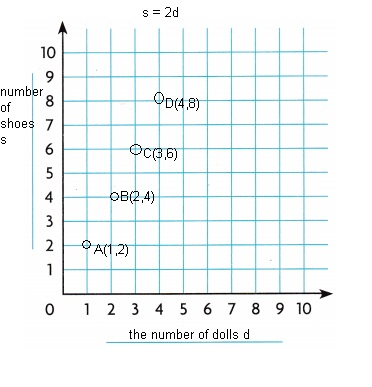The numbers on x – axis is number of dolls d and y- axis is the output number of shoes s as s =2d, so the number pair represented are A(1,2), B(2,4), C(3,6) and D(4,8), Explanation: Given each doll needs 2 shoes. The rule is s = 2d where d is the number of dolls and s is the number of shoes Used the rule to show a pattern on the graph. STEP 1: Labeled the horizontal number line to show the input is the number of dolls as d, Labeled the vertical number line to show the output is the number of shoes s, STEP 2: Wrote a number pair and graphed it above, The input d times 2 is equal to the output s. The number pair is (1,2), STEP 3: Graphed several number pairs as Moved right 1 more units from 0 we get 1 then moved up 1X 2 = 2 units, So we get A(1,2), Moved right 1 more units from 1 we get 2 then moved up 2 x 2 = 4 units, we get B(2,4), Moved right 1 more units from 2 we get 3 then moved up 3 X 3 = 9 units, So we get C(3,9), Moved right 1 more units from 3 we get 4 then moved up 4 X 3 = 12 units, So we get D(4,12), The numbers in the number pair represents A(1,3), B(2,6), C(3,9) and D(4,12) respectively. Question 2. The score for guessing the correct word is 4 and the number of letters in the word. The rule is s = 4 + l where l is the number of letters and s is the score.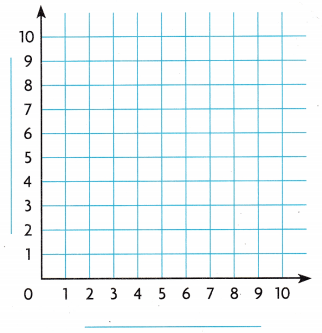Answer:The numbers on x – axis is number of letters l and y- axis is the output score s as s =4 +l, so the number pair represented are A(1,5), B(2,6), C(3,7) and D(4,8), Explanation: Given the score for guessing the correct word is 4 and the number of letters in the word. The rule is s = 4 + l where l is the number of letters and s is the score. Used the rule to show a pattern on the graph. STEP 1: Labeled the horizontal number line to show the input is the number of letters as l, Labeled the vertical number line to show the output is the score s, STEP 2: Wrote a number pair and graphed it above, The input l times plus 4 is equal to the output s. The number pair is (1,5), STEP 3: Graphed several number pairs as Moved right 1 more units from 0 we get 1 then moved up 1+ 4 = 5 units, So we get A(1,5), Moved right 1 more units from 1 we get 2 then moved up 2 + 4 = 6 units, we get B(2,6), Moved right 1 more units from 2 we get 3 then moved up 3 + 4 = 7 units, So we get C(3,7), Moved right 1 more units from 3 we get 4 then moved up 4 + 4 = 8 units, So we get D(4,8), The numbers in the number pair represents A(1,5), B(2,6), C(3,7) and D(4,8) respectively. Problem Solving Question 3. Write Math Explain how you can plot a point on the graph to represent a number pair. Answer: A point is named by its ordered pair of the form of (x, y). The first number corresponds to the x-coordinate and the second to the y-coordinate. To graph a point, We draw a dot at the coordinates that corresponds to the ordered pair. It’s always a good idea to start at the origin. Explanation: The numbers on a coordinate grid are used to locate points. Each point can be identified by an ordered pair of numbers that is, a number on the x-axis called an x-coordinate, and a number on the y-axis called a y-coordinate. Ordered pairs are written in parentheses (x-coordinate, y-coordinate). The origin is located at (0,0). Question 4. H.O.T. Write Math Explain how the first point in your graph for Exercise 2 would change if the rule changes to s = 5 + l. Answer: If the first point in my graph for for Exercise 2 would change if the rule changes to s = 5 + l. The numbers on x – axis is number of letters l and y- axis is the output score s as s =5 +l, so the number pairs represented will changes to A(1,6), B(2,7), C(3,8) and D(4,9), Explanation: If the first point in my graph for for Exercise 2 would change if the rule changes to s = 5 + l. The numbers on x – axis is number of letters l and y- axis is the output score s as s =5 +l, so the number pairs represented will changes to Moved right 1 more units from 0 we get 1 then moved up 1+ 5 = 6 units, So we get A(1,6), Moved right 1 more units from 1 we get 2 then moved up 2 + 5 = 7 units, we get B(2,7), Moved right 1 more units from 2 we get 3 then moved up 3 + 5 = 8 units, So we get C(3,8), Moved right 1 more units from 3 we get 4 then moved up 4 + 5 = 9 units, So we get D(4,9), The numbers in the number pair represents will changes to A(1,6), B(2,7), C(3,8) and D(4,9) respectively. Problem Solving Question 5. H.O.T. Multi-Step Rita uses red and blue ribbons in a design. The length of the blue ribbon, b, is always 3 inches greater than the length of the red ribbons, r. Write a rule and plot 4 points on the graph to show the pattern.Answer:Rule: b= 3 + r The numbers on x – axis is red ribbons and y- axis is the output is blue ribbons as b = 3 + r, so the number pair represented are A(1,4), B(2,5), C(3,6) and D(4,7), Explanation: Given Rita uses red and blue ribbons in a design. The length of the blue ribbon, b, is always 3 inches greater than the length of the red ribbons, r. Wrote a rule and plotted 4 points on the graph to show the pattern above as b = 3 + r, if r =1 then b = 3 + 1 = 4, if r =2 then b = 3 + 2 = 5, if r = 3 then b = 3 + 3 = 6 and if r = 4 then b = 3 + 4 = 7, so the number pair represented on graph are A(1,4), B(2,5), C(3,6) and D(4,7). Question 6. H.O.T. Multi-Step Mina uses green and red ribbons for her design. The length of the green ribbons, g, is always twice the length of the red ribbons, r. Write a rule to describe Mina’s design and plot 4 points on the graph to show the pattern.Answer:Rule: g= 2 X r The numbers on x – axis is red ribbons and y- axis is the output is green ribbons as g = 2 X r, so the number pair represented are A(1,2), B(2,4), C(3,6) and D(4,8), Explanation: Given Mina uses green and red ribbons for her design. The length of the green ribbons, g, is always twice the length of the red ribbons, r. Wrote a rule to describe Mina’s design and plotted 4 points on the graph to show the pattern above Rule: g = 2 X r, if r =1 then g = 2 X 1 = 2, if r =2 then g = 2 X 2 = 4, if r = 3 then g = 2 X 3 = 6 and if r = 4 then g = 2 x 4 = 8, so the number pair represented on graph are A(1,2), B(2,4), C(3,6) and D(4,8). Daily Assessment Task Fill in the bubble completely to show your answer. Question 7. A recipe for carrot juice uses the formula j = 6c, where j is the amount of juice in ounces and c is the number of pounds of carrots needed. How many pounds of carrots are needed for a 30-ounce glass of carrot juice? (A) 5 pounds (B) 24 pounds (C) 180 pounds (D) 36 pounds Answer: (C) 180 pounds Explanation: Given a recipe for carrot juice uses the formula j = 6c, where j is the amount of juice in ounces and c is the number of pounds of carrots needed. Pounds of carrots are needed for a 30-ounce glass of carrot juice is 6 X 30 = 180 pounds. Question 8. Harrison uses the rule y = x + 5 to complete a table and make a graph. Which number pair will be on the graph?(A) (6, 1) (B) (4, 8) (C) (5, 0) (D) (4, 9) Answer: (D) (4, 9), Explanation: Given Harrison uses the rule y = x + 5 to complete a table and make a graph. so number pair will be on the graph are if x = 3 then y = 3 + 5 = 8, (3,8), if x = 4 then y = 4 + 5 = 9, (4,9), if x = 5 then y = 5 + 6 = 10, (5,10), So number pair (D) (4,9) will be on the graph. Question 9. Multi-Step The rule d = 12t shows the cost in dollars, d, for the number of movie tickets, t. Which two points could be on the graph? (A) (0, 12) and (36, 3) (B) (1, 11) and (2, 24) (C) (0, 0) and (3, 36) (D) (0, 12) and (3, 36) Answer: (C) (0, 0) and (3, 36), Explanation: The rule d = 12t shows the cost in dollars, d, for the number of movie tickets t , 1. we will check with the given options if we take option A the second points t is 36 then d should be 12 X 36 = 432 ≠ 3 which will not match with the rule, 2. If t is 1 then d= 12 X 1 = 12 ≠ 11 so (B) will not match, 3. if t = 0 then d = 12 X 0 = 0 and if t = 3 then d = 12 X 3 = 36 which matches with (C), 4. Now we will check with (D) if t= 0 then d = 12 X 0 =0 ≠ 12 so (D) will not match, therefore the correct match is (C) (0, 0) and (3, 36). Texas Test Prep Question 10. Lamar uses the rule s = 7g to show the number of snacks he needs, s, for the number of guests at his party, g. Which number pair shows the number of snacks needed for 4 guests? (A) (4, 28) (B) (1, 8) (C) (4, 14) (D) (28, 4) Answer: (A) (4, 28), Explanation: Given Lamar uses the rule s = 7g to show the number of snacks he needs, s for the number of guests at his party g. So the number pair that shows the number of snacks needed for 4 guests is if g = 4 then s = 7 X 4 = 28 means number pair is (4,28) which matches with (A). ### Texas Go Math Grade 5 Lesson 10.4 Homework and Practice Answer Key Use the rule to graph a pattern. Question 1. The cost for printing pages at a print shop is a$5 processing fee and $1 for each page. The rule is c = 5 + p, where p is the number of pages and c is the total cost.Answer: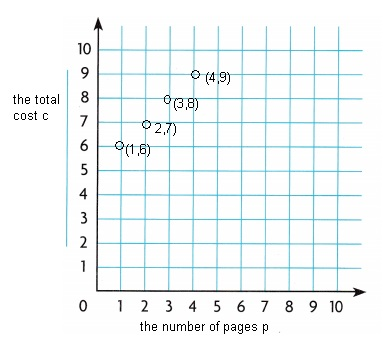Rule : c = 5 + p, Explanation: Given the cost for printing pages at a print shop is a$5 processing fee and $1 for each page. The rule is c = 5 + p, where p is the number of pages and c is the total cost, the 4 points on the graph to show the pattern above if p =1 then c = 5 + 1 = 6, (1,6) if p =2 then c = 5 + 2 = 7, (2,7) if p = 3 then c = 5 + 3 = 8, (3,8) and if p = 4 then c = 5 + 4 = 9, (4,9) so the number pair represented on graph are (1,6), (2,7), (3,8) and (4,9). Question 2. Mario uses craft sticks to form triangles. The rule is c = 3t, where c represents the number of craft sticks and t represents the number of triangles.Answer: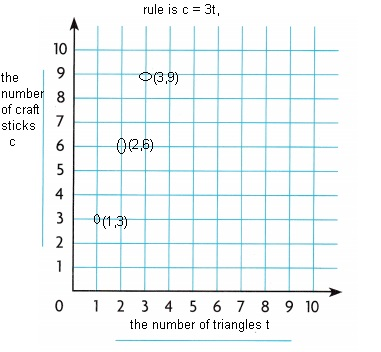Explanation: Given Mario uses craft sticks to form triangles. The rule is c = 3t, where c represents the number of craft sticks and t represents the number of triangles. the 3 points on the graph to show the pattern above if t =1 then c = 3 x 1 = 3, (1,3) if t =2 then c = 3 X 2 = 6, (2,6) and if t = 3 then c = 3 X 3 = 9, (3,9) so the number pair represented on graph are (1,3), B(2,6) and C(3,9). Problem Solving Use the graph for 3-4.Question 3. A department store offers free samples of a 2-ounce container of lotion with every fragrance purchase. Write a rule to describe the number of ounces of product for each purchase. Plot 3 points on the graph to show the pattern. Use t for the total number of ounces and f for the ounces of fragrance. Answer:Rule to describe the number of ounces of product for each purchase is t = f + 2, Explanation: Given a department store offers free samples of a 2-ounce container of lotion with every fragrance purchase. Using t for the total number of ounces and f for the ounces of fragrance. Wrote a rule to describe the number of ounces of product for each purchase as t = f + 2 Plotted 3 points on the graph to show the pattern as if f = 1 then t = 1 + 2 = 3, (1,3), if f = 2 then t = 2 + 2 = 4, (2,4) and if f =3 then t = 3 + 2 = 5, (3,5) respectively. Question 4. Clara buys 6 ounces of fragrance on Monday and returns to buy another 4 ounces of fragrance on Friday. How many ounces of fragrance will she have in all? Answer: Clara will have 10 ounces of fragrance in all, Explanation: Given Clara buys 6 ounces of fragrance on Monday and returns to buy another 4 ounces of fragrance on Friday. So number of ounces of fragrance will she have in all is 6 + 4 = 10 ounces of fragrance. Lesson Check Fill in the bubble completely to show your answer. Question 5. Duncan uses the rule b = 4a to complete a table and make a graph. Which number pair will be on the graph?(A) (3, 12) (B) (3, 7) (C) (4, 8) (D) (12, 3) Answer: (A) (3, 12) number pair will be on the graph, Explanation: Given Duncan uses the rule b = 4a to complete the table and make a graph, so number pair on graph will be if a = 2, b= 4 X 2 = 8, (2,8), if a = 3, b = 4 X 3 = 12, (3, 12), if a = 4, b= 4 X 4 = 16, (4, 16) and if a = 5, b = 4 X 5 = 20, (5,20) so from given choices the matching pair is (A) (3, 12). Question 6. The online music store charges a$6 fee for becoming a member and
$1 to download each song. Noriko uses the rule c = 6 + s to calculate her cost. Which number pair shows the cost of 5 songs? (A) (5, 6) (B) (5, 11) (C) (5, 5) (D) (1, 5) Answer: Number pair shows the cost of 5 songs is (B) (5,11), Explanation: Given the online music store charges a$6 fee for becoming a member and
\$1 to download each song. Noriko uses the rule c = 6 + s to calculate her cost.
So number pair shows the cost of 5 songs is c = 6 + 5 = 11 means (5,11).

Question 7.
The camp counselors use the equation c = 8g where c is the number of
campers and g is the number of groups. Which number pair will not be a
point on the graph of the equation?
(A) (0, 0)
(B) (3, 24)
(C) (2, 16)
(D) (8, 1)
(D) (8, 1) number pair will not be a point on the graph of the equation,

Explanation:
Given the camp counselors use the equation c = 8g,
where c is the number of campers and g is the number of groups,
now we will see which number pair will not be a point on the graph of the equation,
first (A) (0,0) means if g = 0 then c = 8 X 0 =0, so (0,0) will be on the graph,
now (B) (3,24) means if g = 3 then c = 8 X 3 = 24, so (3,24) will be on the graph,
now (C) (2,16) means if g =2 then c = 8 X 2 = 16, so(2, 16) will be on the graph,
and finally (D) (8,1) means if g = 8 then c = 8 X 8 = 64 ≠ 1 will be not on the graph,
therefore (D) (8, 1) number pair will not be a point on the graph of the equation.

Question 8.
Multi-Step The rule j = 16p shows the number of ounces j for
each p pint of orange juice. Which two points will be on the graph?
(A) (2, 32) and (3, 48)
(B) (0, 16) and (1, 32)
(C) (2, 32) and (4, 40)
(D) (1, 8) and (2, 16)
(A) (2, 32) and (3, 48),

Explanation:
The rule j = 16p shows the number of ounces j for
each p pint of orange juice,
1. we will check with the given options if we take option A the
if p is 2 then j is 16 X 2 = 32 and second point if p is 3 then
j is 16 X 3 = 48 which matches the rule,
2. If p is 0 then j= 16 X 0 = 0 ≠ 16 so (B) will not match,
3. if p = 2 then j = 16 X 2 = 32 and if p = 4 then j = 16 X  4 = 64 ≠ 40
so (C) will not match,
4. Now we will check with (D) if p= 1 then j = 16 X 1 =16 ≠ 8
so (D) will not match,
therefore  the correct  match is (A) (2, 32) and (3, 48).

Question 9.
Multi-Step The rule g = 5 + y shows the relationship of yellow, y, and
green, g, split peas in Wendy’s soup recipe.
Which point on the grid does NOT show the relationship?(A) (5, 10)
(B) (20, 25)
(C) (15, 10)
(D) (15, 20)
(C) (15, 10) point on the grid does NOT show the relationship,

Explanation:
Given the rule g = 5 + y shows the relationship of yellow, y, and
green, g, split peas in Wendy’s soup recipe
the points on the grid are 1. (5,10), 2. (15,10), 3. (15,20), 4. (20,25),
now checking the rule for each
1. (5,10) means if y = 5 then g = 5 + 5 = 10 is correct,
2. (15,10) means if y = 15 then g = 15 + 5 = 20 ≠ 10 which is incorrect,
3. (15,20) means if y = 15 then g = 15 + 5 = 20 is correct,
4. (20,25) means if y = 20 then g = 20 + 5 = 25 which is correct,
therefore, (C) (15, 10) point on the grid does NOT show the relationship,

Scroll to Top